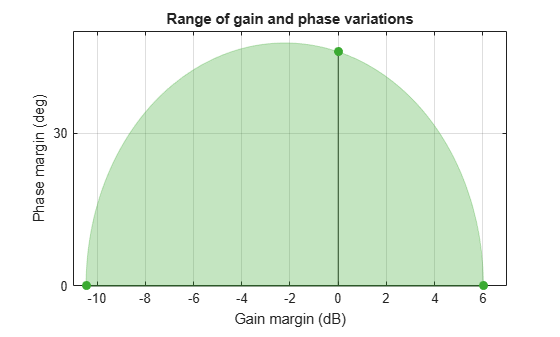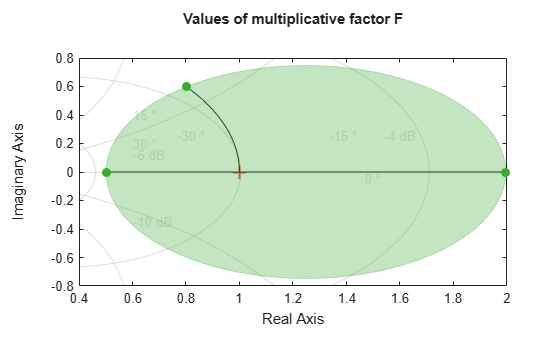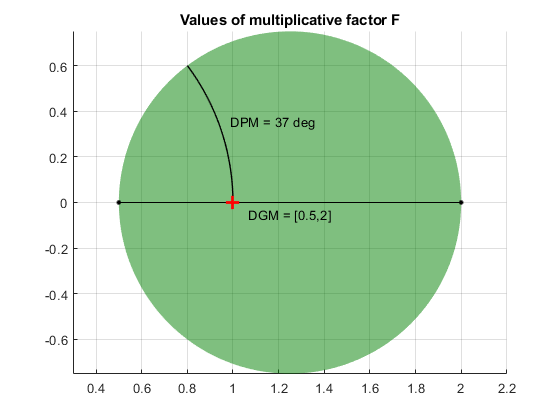# getDPM

Disk-based phase variation corresponding to disk-based gain variation

## Syntax

``DPM = getDPM(DGM)``
``DPM = getDPM(GM)``

## Description

example

````DPM = getDPM(DGM)` returns the disk-based phase variation corresponding to disk-based gain variation `DGM`. In the model used by `umargin`, gain and phase variation are represented as a multiplicative factor F(s) taking values in a disk centered on the real axis. The disk described by its real-axis intercepts `DGM` = `[gmin,gmax]`, which represent the relative amount of gain variation around the nominal value F = 1. Because the disk is complex-valued, the disk described by `DGM` also represents a certain amount of phase variation, `DPM`. For more information, see `getDGM`.```

example

````DPM = getDPM(GM)` is the same as `getDPM([1/GM,GM])`. This syntax returns the disk-based phase variation range corresponding to a gain that can increase or decrease by a factor `GM`.```

## Examples

collapse all

Find the disk-based phase margin which corresponds to the disk-based gain margin with variations in the range `[0.3,2]`.

```DGM = [0.3,2]; DPM = getDPM(DGM)```
```DPM = 1×2 -45.9208 45.9208 ```

`DGM = [0.3,2`] describes a disk of multiplicative gain and phase uncertainty in which the gain can vary from 0.3 times the nominal value to twice the nominal value (at nominal phase). Visualize the disk.

`diskmarginplot(DGM,'disk')`The disk also describes phase variations of about ±46°. `getDPM` returns this phase range as `DPM`, which is the range in which the modeled phase can vary at nominal gain. Visualize the range of simultaneous gain and phase variations captured in the disk described by `DGM = [0.3,2]` and `DPM = [-45.92 45.92]`.

`diskmarginplot(DGM)`Find the disk-based phase margin corresponding to the gain variation of ±6 dB, or a factor of 2 in either direction.

```GM = db2mag(6); DPM = getDPM(GM)```
```DPM = 1×2 -36.7611 36.7611 ```

For a scalar input `GM`, `getDPM(GM)` is same as `getDPM([1/GM,GM])`.

`DPM = getDPM([1/GM,GM])`
```DPM = 1×2 -36.7611 36.7611 ```

For the given gain variation of ±6 dB, the corresponding disk models the phase variation range of about ±36.8° at nominal gain. Visualize the disk and the combined gain and phase variations it represents.

`diskmarginplot([1/GM,GM],'disk')``diskmarginplot([1/GM,GM])`## Input Arguments

collapse all

Range of relative gain variation, specified as a two-element vector of the form `[gmin,gmax]`, where `gmin` < 1 and `gmax` > 1. For instance, `DGM = [0.8 1.5]` represents a gain that can vary between 80% and 150% of its nominal value (that is, change by a factor between 0.8 and 1.5). `gmin` can be negative, defining a range of relative gain variation that includes a change in sign.

`DGM` represents that the disk intersects real axis in the interval `[gmin,gmax]`, where `gmin` < 1 and `gmax` > 1. For instance, the following plot shows a disk characterized by `DGM = [0.5,2]`.You can obtain `DGM` from desired gain and phase variations (or margins) using `getDGM`. The `GainMargin` field of the output structures of the `diskmargin` command is also a disk-based gain range of this form. .

To get `DPM` corresponding to multiple gain ranges at once, specify is a two-column matrix of form `[gmin1,gmax1;...;gminN,gmaxN]`.

Amount of gain increase or decrease, specified as a real scalar or column vector.

• If `GM` is a real scalar, then `getDPM` returns the disk-based phase variation corresponding to a symmetric gain variation of `[1/GM,GM]`. For instance, `GM` = 2 specifies a gain that can increase or decrease by a factor of 2.

• If `GM` is a vector of form `[GM1;...;GMN]`, then the function returns disk-based phase variations corresponding to each range `[1/GM1,GM1;...;1/GMN,GMN]`.

## Output Arguments

collapse all

Disk-based phase variation, returned as a two-element vector or a two-column matrix.

The vector `DPM = [-pm,pm]`, represents the relative phase variation amount determined by the geometry of the disk described by `DGM`. For more information, see `getDGM`.

If `DGM` is a two-column matrix containing multiple gain-variation ranges, the function returns a two-column matrix of the form `[-pm1,pm1; ...;-pmN,pmN]`.

### Topics

Introduced in R2020a

## SupportGet trial now